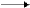Rate low – Influence of concentration

It has already been proven that the rate of a chemical reaction is generally given by the equation   υ = k f (c1,c2,…cn)
Where
f(c1,c2,…cn)
is the cohesion of the reactants concentration and k is a constant which is dependant from all the other factors that influence the rate besides concentration.

For each one of the following reactions it has been proven experimentally that the expression of the rate is the one mentioned below.

N2O52NO2 + 1/2 O2       υ = k[N2O5]

H2 +I22HI                    υ = k[H2][I2]

2NO + O22NO2          υ = k[NO]2[O2]
From the above types it could be deduced the following rule which helps us find the expression of the rate of a chemical reaction.
“ The rate of a chemical reaction is proportional to the active mass of the reactants”

Active mass is defined as the molar per volume concentration of the reactant raised in to the power of an exponent which is equal to the coefficient of the reactant’s concentration.

For the reaction   aA + bBΠ    (are the reactants) the expression of the rate, which is also called rate low, is given by the equation:

υ = k[A]a[B]b

Deviations from the above rule exist in many occasions and are mainly due to the fact that the reaction is taking place in more than one steps.
Example:

For the reaction
CH3CHOCH4 + CO    is experimentally proven that
υ = k[CH3CHO]3/2          and not      υ = k[CH3CHO]

Sometimes the question that appears is which is the correct way to quote a chemical reaction in order to characterize it as simple. For instance in the formation of HI the question that arises is whether the reaction should be written in the form

H2 + I22HI    (the corresponding type is  υ = k[H2][I2] )
or in the form
1/2 H
2 +1/2 I2HI (the corresponding type υ = k[H2]1/2[I2]1/2).
The answer in this case is: As far as the chapter of chemical kinetics is concerned, the way of quoting the reaction is not an issue because the two different ways mentioned above express two totally different chemical reactions.

Specifically, the reaction

H2 + I22HI   indicates that one particular

H2  molecule reacts with one particular I2 molecule to form HI, while the reaction 1/2 H2 +1/2 I2HI  indicates that primarily dissociation of the  I2 and H2 molecules into atoms takes place which then react in their turn and produce HI.
Experimental data proves that in the formation of HI the reaction that takes place is: H
2 + I22HI  and the rate low that applies is  υ = k[H2][I2] .

Presence of solid substances:

The solid substances are excluded from the expression of the rate low because they react only with the surface that gets in touch with the other reactants and not with their whole quantity.
Thus, when solid substances react with gases, liquids or dissolved substances the rate of the reaction depends upon all the other substances but the solid ones.
For instance, for the reaction

C(s) + H2O(g)CO(g) + H2(g)   the rate that applies is   υ= k[H2O]